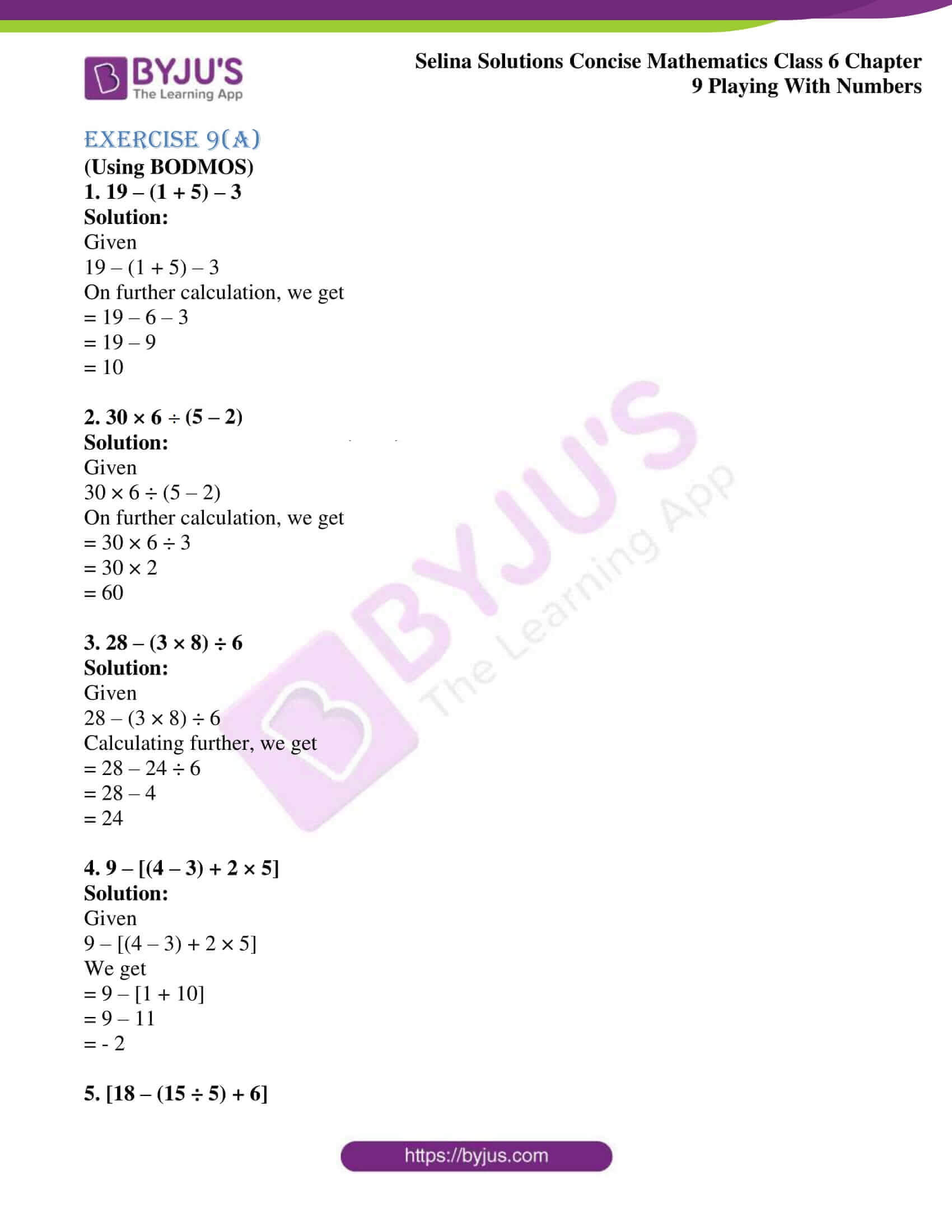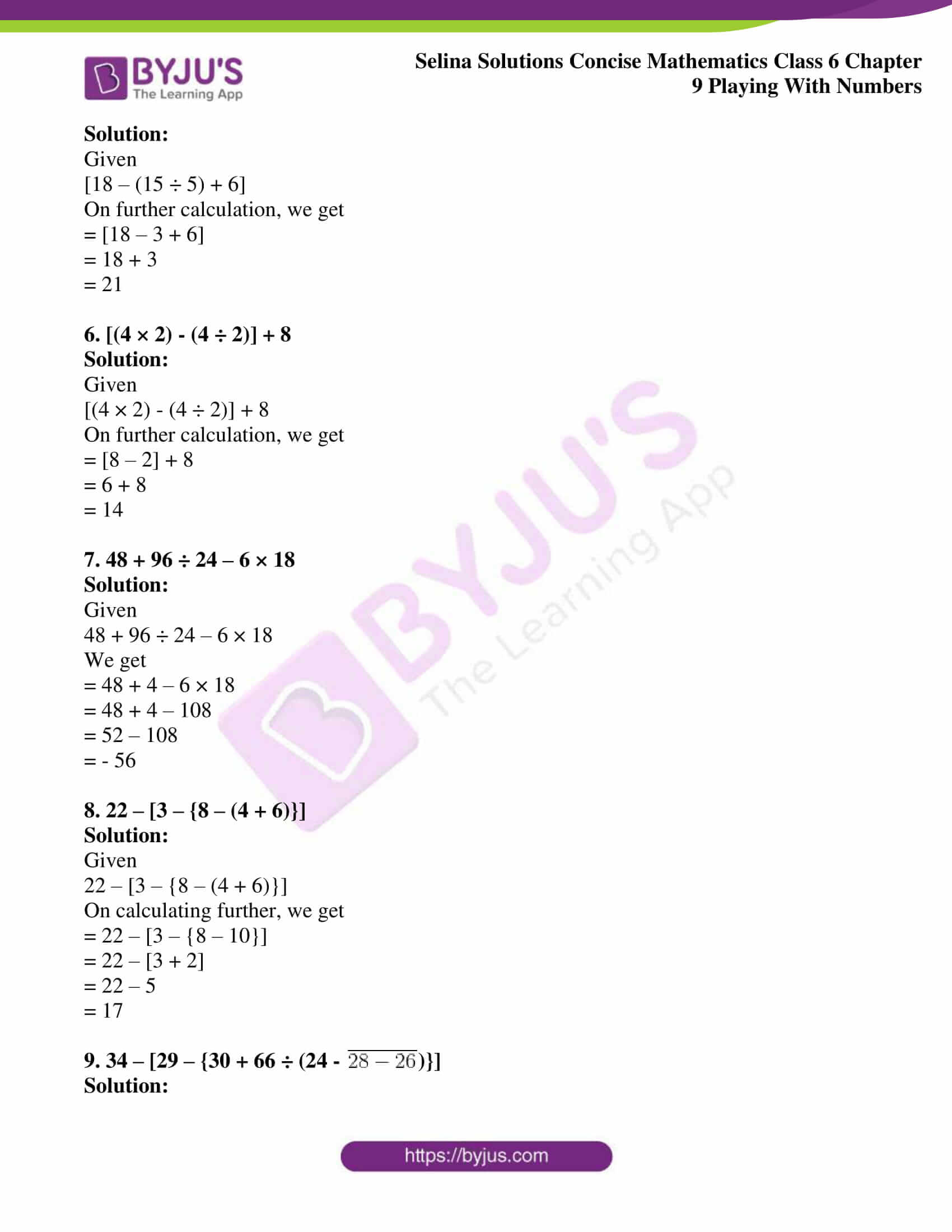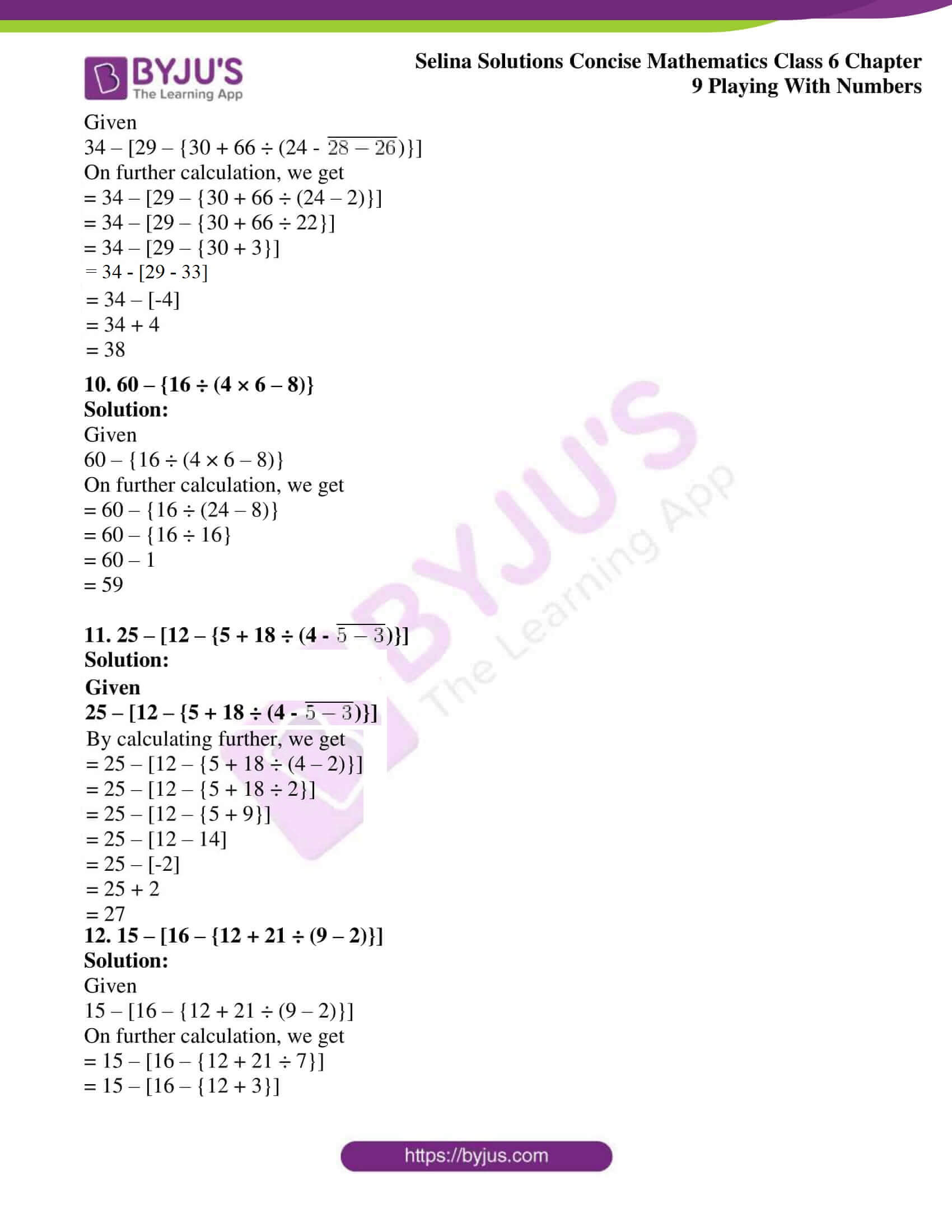# Selina Solutions Concise Mathematics Class 6 Chapter 9: Playing With Numbers Exercise 9(A)

Selina Solutions Concise Mathematics Class 6 Chapter 9 Playing With Numbers Exercise 9(A) helps to solve the problems using BODMAS, as per the steps mentioned in the textbook. Expert teachers at BYJU’S have prepared solutions with the intention to help students in boosting their exam preparation. Frequent practice of Selina Solutions helps students to obtain a better idea of solving tricky questions without any obstacles. These Selina Solutions are the key source among students in clearing their doubts during the examination. The PDF of Selina Solutions Concise Mathematics Class 6 Chapter 9 Playing With Numbers Exercise 9(A) are available, from the below links for free download

## Selina Solutions Concise Mathematics Class 6 Chapter 9: Playing With Numbers Exercise 9(A) Download PDF### Access other exercises of Selina Solutions Concise Mathematics Class 6 Chapter 9: Playing With Numbers

Exercise 9(B) Solutions

Exercise 9(C) Solutions

### Access Selina Solutions Concise Mathematics Class 6 Chapter 9: Playing With Numbers Exercise 9(A)

#### Exercise 9(A)

(Using BODMOS)

1. 19 – (1 + 5) – 3

Solution:

Given

19 – (1 + 5) – 3

On further calculation, we get

= 19 – 6 – 3

= 19 – 9

= 10

2. 30 × 6 ÷ (5 – 2)

Solution:

Given

30 × 6 ÷ (5 – 2)

On further calculation, we get

= 30 × 6 ÷ 3

= 30 × 2

= 60

3. 28 – (3 × 8) ÷ 6

Solution:

Given

28 – (3 × 8) ÷ 6

Calculating further, we get

= 28 – 24 ÷ 6

= 28 – 4

= 24

4. 9 – [(4 – 3) + 2 × 5]

Solution:

Given

9 – [(4 – 3) + 2 × 5]

We get

= 9 – [1 + 10]

= 9 – 11

= – 2

5. [18 – (15 ÷ 5) + 6]

Solution:

Given

[18 – (15 ÷ 5) + 6]

On further calculation, we get

= [18 – 3 + 6]

= 18 + 3

= 21

6. [(4 × 2) – (4 ÷ 2)] + 8

Solution:

Given

[(4 × 2) – (4 ÷ 2)] + 8

On further calculation, we get

= [8 – 2] + 8

= 6 + 8

= 14

7. 48 + 96 ÷ 24 – 6 × 18

Solution:

Given

48 + 96 ÷ 24 – 6 × 18

We get

= 48 + 4 – 6 × 18

= 48 + 4 – 108

= 52 – 108

= – 56

8. 22 – [3 – {8 – (4 + 6)}]

Solution:

Given

22 – [3 – {8 – (4 + 6)}]

On calculating further, we get

= 22 – [3 – {8 – 10}]

= 22 – [3 + 2]

= 22 – 5

= 17

9. 34 – [29 – {30 + 66 ÷ (24 –

$$\begin{array}{l}\overline{28 – 26}\end{array}$$
)}]

Solution:

Given

34 – [29 – {30 + 66 ÷ (24 –

$$\begin{array}{l}\overline{28 – 26}\end{array}$$
)}]

On further calculation, we get

= 34 – [29 – {30 + 66 ÷ (24 – 2)}]

= 34 – [29 – {30 + 66 ÷ 22}]

= 34 – [29 – {30 + 3}]

= 34 – [29 – 33]

= 34 – [-4]

= 34 + 4

= 38

10. 60 – {16 ÷ (4 × 6 – 8)}

Solution:

Given

60 – {16 ÷ (4 × 6 – 8)}

On further calculation, we get

= 60 – {16 ÷ (24 – 8)}

= 60 – {16 ÷ 16}

= 60 – 1

= 59

11. 25 – [12 – {5 + 18 ÷ (4 –

$$\begin{array}{l}\overline{5 – 3}\end{array}$$
)}]

Solution:

Given

25 – [12 – {5 + 18 ÷ (4 –

$$\begin{array}{l}\overline{5 – 3}\end{array}$$
)}]

By calculating further, we get

= 25 – [12 – {5 + 18 ÷ (4 – 2)}]

= 25 – [12 – {5 + 18 ÷ 2}]

= 25 – [12 – {5 + 9}]

= 25 – [12 – 14]

= 25 – [-2]

= 25 + 2

= 27

12. 15 – [16 – {12 + 21 ÷ (9 – 2)}]

Solution:

Given

15 – [16 – {12 + 21 ÷ (9 – 2)}]

On further calculation, we get

= 15 – [16 – {12 + 21 ÷ 7}]

= 15 – [16 – {12 + 3}]

= 15 – [16 – 15]

= 15 – 1

= 14